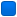###Author Topic: Curious behavior of compiled program (if jumping out of while loop)  (Read 694 times)

####sgraffe

• Member
•• Posts: 20##### Curious behavior of compiled program (if jumping out of while loop)
« on: March 10, 2018, 05:27:40 pm »
Hello, all:

I am getting strange results when debugging this code:
(The logic of the code is wrong because I am just modifying it)

Code: [Select]
` while(projTable->readNextRecord(projTable) != EOF) { int thisProjectID = atoi(projTable->readField(projTable, proj_id)); if (projectID_ == 0) { projectID_ == thisProjectID; break; } if (thisProjectID == projectID_) break; } if (projectID_ == 0) return setErrStatus(projectNotFound);`At the beginning, projectID_ is zero. When the program reaches the first if (if (projectID_ == 0))
it jumps out of the loop to the line with the return.

A new version of the code:
Code: [Select]
` bool found = false; int thisProjectID = 0; while(!found && projTable->readNextRecord(projTable) != EOF) { thisProjectID = atoi(projTable->readField(projTable, proj_id)); if (projectID_ == 0) { projectID_ == thisProjectID; found = true; break; } if (thisProjectID == projectID_) { found = true; break; } } if (! found) if (projectID_ == 0)`
In this case, from the first if, it jumps inside the second if (if (thisProjectID == projectID_)), to the break statement.

My final working version is this (it works ok):
Code: [Select]
` bool found = false; int thisProjectID = 0; while(!found && projTable->readNextRecord(projTable) != EOF) { thisProjectID = atoi(projTable->readField(projTable, proj_id)); if (projectID_ == 0) found = true; else found = thisProjectID == projectID_; } if (!found) return setErrStatus(projectNotFound);`
Can anybody find an explanation of this behavior?
This is so simple that I am sure I screwed it somewhere.
It looks like the program  does not execute what the source code says.

Thank you for your time,
Simon

####frankie

• Global Moderator
• Member
••• Posts: 1686##### Re: Curious behavior of compiled program (if jumping out of while loop)
« Reply #1 on: March 10, 2018, 10:40:17 pm »
This is due to optimizations. The line:
Code: [Select]
`projectID_ == thisProjectID;`Isn't an assignment, the operator == compares the 2 values, but have no side effects, so the compiler optimizes it out omitting to emit any code for it.
Said that, it happens the following:
• In the first case nothing is executed after the if, and because the next comparison checks for projectID_ equal to zero execution jumps to return.
• In the second case the execution jumps inside the second if because the code, after omission of the null statement, is the same for both if's and compiler optimized using the same code.
• In the last case you removed the null statement making a distinct flow for the different execution paths.

####sgraffe

• Member
•• Posts: 20##### Re: Curious behavior of compiled program (if jumping out of while loop)
« Reply #2 on: March 10, 2018, 11:22:47 pm »
I knew I had screwed it!
But four eyes were needed to find it.
That statement should read
projectID_ = thisProjectID;

and not
projectID_ == thisProjectID;

Thank you!

Simon